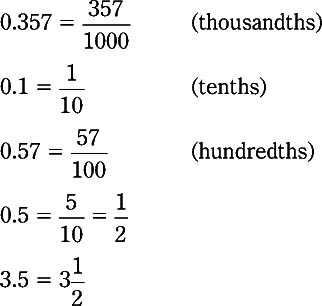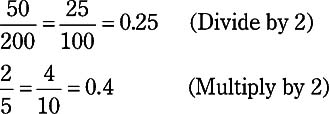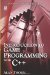# 1.9 Decimal Notation-Damn Those Dots

## 1.9 Decimal Notation-Damn Those Dots

Randolph Churchill, the father of British Prime Minister Winston Churchill, once said when referring to decimals that he "never could make out what those damned dots meant." This section attempts to explain them and decimal notation generally. In a previous section we saw how numbers are grouped into columns by place value, where the number 351 means 3 hundreds, 5 tens, and 1 unit. Each column is a multiple of 10, beginning from the rightmost side. However, so far decimal notation has only been used to express positive whole numbers. Decimals, though, can be used to express fractions and mixed numbers. It does this using a decimal point. These types of fractions are called decimal fractions, as opposed to common fractions, or vulgar fractions, which we explored in previous sections. Here are examples of decimals:

 351.5 471.07 951.3 0.9753

These numbers are not whole numbers, but are mixed or entirely fractional. The numbers to the left of the decimal point are whole numbers, and the numbers to the right represent a fraction. The last number (0.9753) has no whole number part because 0 is to the left of the decimal; this number is not a mixed number, but entirely a fractional.

In short, numbers to the right of the decimal are a fraction; they are the numerator of a fraction and we do not write the denominator. Like all other columns of decimal notation, the base is base 10. Whereas the numbers to the left of the decimal point increase by a multiple of 10 from each column to the left-units, tens, hundreds, thousands-the numbers to the right of the decimal point continue to decrease in each column by a multiple of 10. Each column is tenths, hundredths, thousandths, and so on. For this reason, when we say numbers to the right of the decimal point are the numerator of a fraction, we do not write the denominator because we already know it to be a power of 10. So:### 1.9.1 Converting Vulgar Fractions to Decimals

Given a fraction, convert it into a decimal. If the fraction is over a denominator that is a power of 10, the denominator can be removed and the numerator can be written to the right of the decimal point, such as 3/10 = 0.3. If the fraction is an improper fraction, it should first be written as a mixed number; any whole numbers are written to the left of the decimal point, and the fractional portion appears after the decimal point. If, however, the denominator of a fraction is not a power of 10 such as ¾, then how should it be converted?

Converting a fraction into one with a denominator of a power of 10 is similar to finding the LCM between two fractions. The fraction must be multiplied or reduced into an equivalent fraction with a power of 10 denominator. Here are some examples: 50Note Using a calculator, the standard method for changing a fraction into a decimal is to divide the numerator by the denominator. For example, to find the decimal equivalent of ½, try dividing 1 by 2, and the result will be 0.5.Introduction to Game Programming with C++ (Wordware Game Developers Library)
ISBN: 1598220322
EAN: 2147483647
Year: 2007
Pages: 225
Authors: Alan Thorn

Similar book on Amazon

flylib.com © 2008-2017.
If you may any questions please contact us: flylib@qtcs.net